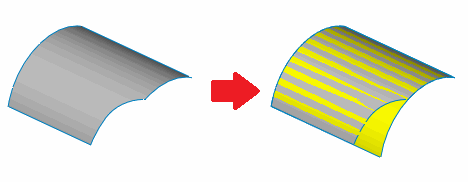# Untrim face or surface with temporary geometry using SOLIDWORKS API

This VBA example restores the surface of the selected face by performing the untrim operation.

This command is similar to the Untrim Surface feature in the Feature Manager, but it performs the operation using temporary bodies instead of features.

Copied surface used in operations is infinite and needs to be trimmed in order to form a face. Required boundary is calculated by evaluating the maximum and minimum values of the UV of the input face.UV bounds of face

Iso curves are used to extract the curve at the specified boundary UV of the face. The calculated curve is infinite and needs to be trimmed in the corners to form the closed loop before the surface can be trimmed and converted into the body.

Select any face and run the macro. The resulting surface is displayed in the graphics area and macro stops execution. Once continued - the preview is hidden.Input surface and untrimmed result

```Dim swApp As SldWorks.SldWorks

Sub main()

Set swApp = Application.SldWorks

Dim swModel As SldWorks.ModelDoc2

Set swModel = swApp.ActiveDoc

If Not swModel Is Nothing Then

Dim swFace As SldWorks.Face2

Set swFace = swModel.SelectionManager.GetSelectedObject6(1, -1)

If Not swFace Is Nothing Then

Dim swSurf As SldWorks.Surface
Set swSurf = swFace.GetSurface

Set swSurf = swSurf.Copy

Dim vUvBounds As Variant
vUvBounds = swFace.GetUVBounds()

Dim swCurves(3) As SldWorks.Curve

Dim uMin As Double
Dim uMax As Double
Dim vMin As Double
Dim vMax As Double

uMin = CDbl(vUvBounds(0))
uMax = CDbl(vUvBounds(1))
vMin = CDbl(vUvBounds(2))
vMax = CDbl(vUvBounds(3))

Dim uMinvMinPt As Variant
Dim uMinvMaxPt As Variant
Dim uMaxvMinPt As Variant
Dim uMaxvMaxPt As Variant

uMinvMinPt = swSurf.Evaluate(uMin, vMin, 0, 0)
uMinvMaxPt = swSurf.Evaluate(uMin, vMax, 0, 0)
uMaxvMinPt = swSurf.Evaluate(uMax, vMin, 0, 0)
uMaxvMaxPt = swSurf.Evaluate(uMax, vMax, 0, 0)

Const V As Boolean = True
Const U As Boolean = False

Set swCurves(0) = swSurf.MakeIsoCurve2(U, uMin)
Set swCurves(0) = swCurves(0).CreateTrimmedCurve2(uMinvMinPt(0), uMinvMinPt(1), uMinvMinPt(2), uMinvMaxPt(0), uMinvMaxPt(1), uMinvMaxPt(2))

Set swCurves(1) = swSurf.MakeIsoCurve2(V, vMin)
Set swCurves(1) = swCurves(1).CreateTrimmedCurve2(uMinvMinPt(0), uMinvMinPt(1), uMinvMinPt(2), uMaxvMinPt(0), uMaxvMinPt(1), uMaxvMinPt(2))

Set swCurves(2) = swSurf.MakeIsoCurve2(U, uMax)
Set swCurves(2) = swCurves(2).CreateTrimmedCurve2(uMaxvMinPt(0), uMaxvMinPt(1), uMaxvMinPt(2), uMaxvMaxPt(0), uMaxvMaxPt(1), uMaxvMaxPt(2))

Set swCurves(3) = swSurf.MakeIsoCurve2(V, vMax)
Set swCurves(3) = swCurves(3).CreateTrimmedCurve2(uMinvMaxPt(0), uMinvMaxPt(1), uMinvMaxPt(2), uMaxvMaxPt(0), uMaxvMaxPt(1), uMaxvMaxPt(2))

Dim swUntimSurfBody As SldWorks.Body2

Set swUntimSurfBody = swSurf.CreateTrimmedSheet5(swCurves, False, 0.00001)

swUntimSurfBody.Display3 swModel, RGB(255, 255, 0), swTempBodySelectOptions_e.swTempBodySelectOptionNone

Stop

Set swUntimSurfBody = Nothing

Else
Err.Raise vbError, , "Select face"
End If

Else
Err.Raise vbError, , "Open the model"
End If

End Sub

```